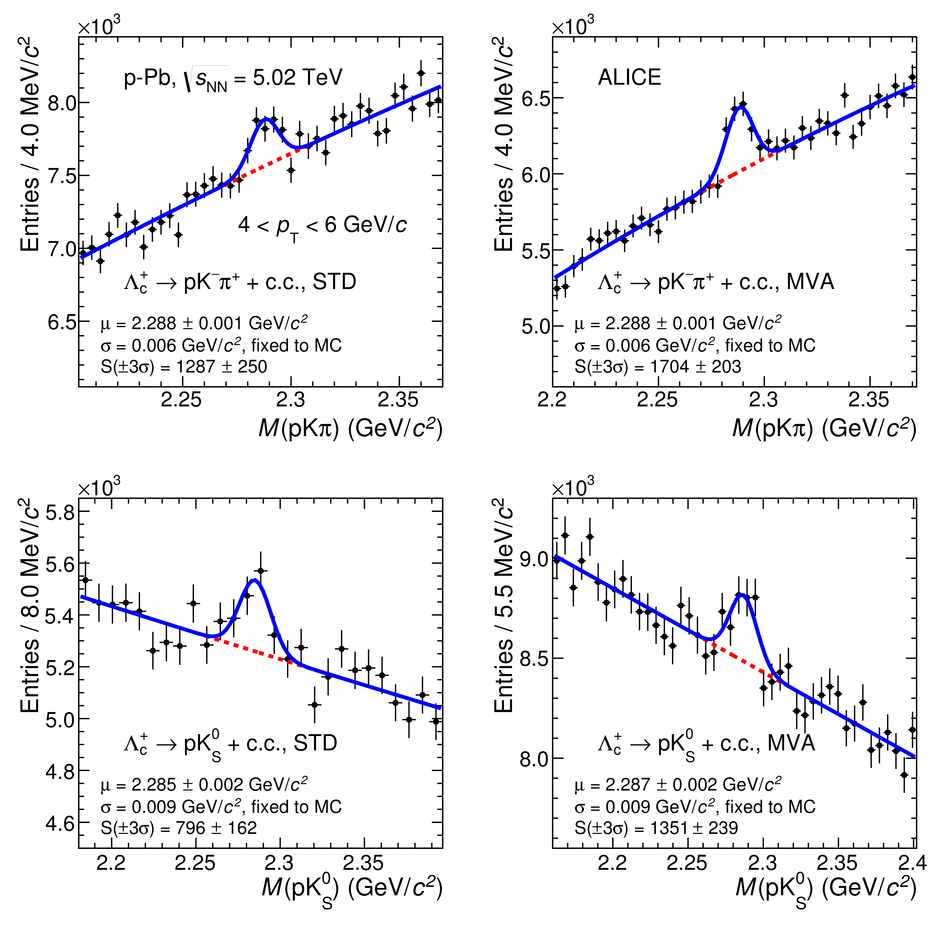# Figure 4

 Invariant-mass distribution of $\Lcplus$ candidates (and charge conjugates) for $4 <$ \pt $< 6$ \gevc in \pPb collisions at \sqrtsNN = 5.02~\tev. The dashed lines represent the fit to the background while the solid lines represent the total fit function. Top-left: $\LctopKpi$ STD analysis, top-right: $\LctopKpi$ MVA, bottom-left: $\LctopKzS$ STD analysis and bottom-right: $\LctopKzS$ MVA.